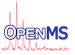OpenMS  2.8.0
GaussFilterAlgorithm Class Reference

This class represents a Gaussian lowpass-filter which works on uniform as well as on non-uniform profile data. More...

#include <OpenMS/FILTERING/SMOOTHING/GaussFilterAlgorithm.h>

Collaboration diagram for GaussFilterAlgorithm:
[legend]

## Public Member Functions

GaussFilterAlgorithm ()
Constructor. More...

virtual ~GaussFilterAlgorithm ()
Destructor. More...

bool filter (OpenMS::Interfaces::SpectrumPtr spectrum)
Smoothes an Spectrum containing profile data. More...

bool filter (OpenMS::Interfaces::ChromatogramPtr chromatogram)
Smoothes an Chromatogram containing profile data. More...

template<typename ConstIterT , typename IterT >
bool filter (ConstIterT mz_in_start, ConstIterT mz_in_end, ConstIterT int_in_start, IterT mz_out, IterT int_out)
Smoothes an two data arrays containing data. More...

void initialize (double gaussian_width, double spacing, double ppm_tolerance, bool use_ppm_tolerance)

## Protected Member Functions

template<typename InputPeakIterator >
double integrate_ (InputPeakIterator x, InputPeakIterator y, InputPeakIterator first, InputPeakIterator last)
Computes the convolution of the raw data at position x and the gaussian kernel. More...

## Protected Attributes

std::vector< double > coeffs_
Coefficients. More...

double sigma_
The standard derivation. More...

double spacing_
The spacing of the pre-tabulated kernel coefficients. More...

bool use_ppm_tolerance_

double ppm_tolerance_

## Detailed Description

This class represents a Gaussian lowpass-filter which works on uniform as well as on non-uniform profile data.

Gaussian filters are important in many signal processing, image processing, and communication applications. These filters are characterized by narrow bandwidths, sharp cutoffs, and low passband ripple. A key feature of Gaussian filters is that the Fourier transform of a Gaussian is also a Gaussian, so the filter has the same response shape in both the time and frequency domains. The coefficientsof the Gaussian-window with lengthare calculated from the gaussian distributionwhererepresents the window area andis the standard derivation.

Note
The wider the kernel width the smoother the signal (the more detail information get lost!). Use a gaussian filter kernel which has approximately the same width as your mass peaks, whereas the gaussian peak width corresponds approximately to 8*sigma.
The data must be sorted according to ascending m/z!

## ◆ GaussFilterAlgorithm()

 GaussFilterAlgorithm ( )

Constructor.

## ◆ ~GaussFilterAlgorithm()

 virtual ~GaussFilterAlgorithm ( )
virtual

Destructor.

## ◆ filter() [1/3]

 bool filter ( ConstIterT mz_in_start, ConstIterT mz_in_end, ConstIterT int_in_start, IterT mz_out, IterT int_out )
inline

Smoothes an two data arrays containing data.

Convolutes the filter and the profile data and writes the results into the output iterators mz_out and int_out.

## ◆ filter() [2/3]

 bool filter ( OpenMS::Interfaces::ChromatogramPtr chromatogram )
inline

Smoothes an Chromatogram containing profile data.

## ◆ filter() [3/3]

 bool filter ( OpenMS::Interfaces::SpectrumPtr spectrum )
inline

Smoothes an Spectrum containing profile data.

## ◆ initialize()

 void initialize ( double gaussian_width, double spacing, double ppm_tolerance, bool use_ppm_tolerance )

## ◆ integrate_()

 double integrate_ ( InputPeakIterator x, InputPeakIterator y, InputPeakIterator first, InputPeakIterator last )
inlineprotected

Computes the convolution of the raw data at position x and the gaussian kernel.

References OpenSwath::norm().

## ◆ coeffs_

 std::vector coeffs_
protected

Coefficients.

## ◆ ppm_tolerance_

 double ppm_tolerance_
protected

## ◆ sigma_

 double sigma_
protected

The standard derivation.

## ◆ spacing_

 double spacing_
protected

The spacing of the pre-tabulated kernel coefficients.

## ◆ use_ppm_tolerance_

 bool use_ppm_tolerance_
protected